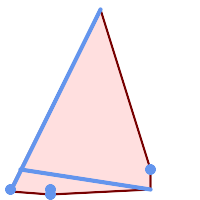## Name

ST_ConvexHull — Computes the convex hull of a geometry.

## Synopsis

`geometry ST_ConvexHull(`geometry geomA`)`;

## Description

Computes the convex hull of a geometry. The convex hull is the smallest convex geometry that encloses all geometries in the input.

One can think of the convex hull as the geometry obtained by wrapping an rubber band around a set of geometries. This is different from a concave hull which is analogous to "shrink-wrapping" the geometries. A convex hull is often used to determine an affected area based on a set of point observations.

In the general case the convex hull is a Polygon. The convex hull of two or more collinear points is a two-point LineString. The convex hull of one or more identical points is a Point.

This is not an aggregate function. To compute the convex hull of a set of geometries, use ST_Collect to aggregate them into a geometry collection (e.g. `ST_ConvexHull(ST_Collect(geom))`.

Performed by the GEOS moduleThis method implements the OGC Simple Features Implementation Specification for SQL 1.1. s2.1.1.3This method implements the SQL/MM specification. SQL-MM 3: 5.1.16This function supports 3d and will not drop the z-index.

## ExamplesConvex Hull of a MultiLinestring and a MultiPoint

```SELECT ST_AsText(ST_ConvexHull(
ST_Collect(
ST_GeomFromText('MULTILINESTRING((100 190,10 8),(150 10, 20 30))'),
ST_GeomFromText('MULTIPOINT(50 5, 150 30, 50 10, 10 10)')
)) );
---st_astext--
POLYGON((50 5,10 8,10 10,100 190,150 30,150 10,50 5))
```

Using with ST_Collect to compute the convex hulls of geometry sets.

```--Get estimate of infected area based on point observations
SELECT d.disease_type,
ST_ConvexHull(ST_Collect(d.geom)) As geom
FROM disease_obs As d
GROUP BY d.disease_type;
```